# Transient Forced Vibration of a Simply-supported Thin Square PlateThis test lets you check analysis results for a simply-supported thin square plate, in the context of a transient dynamic response case. You will use 2D meshes.

This test is used to validate the following attributes:

• 2D shell elements (i.e. the elementary stiffness and mass matrices)

• Transient dynamic response solve algorithms.Reference:

NAFEMS-Glasgow, Benchmark newsletter, Report No. E1261/R002, p.21, February 1989.

##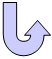Specifications

### Geometry Specifications

 Length: L = 10000 mm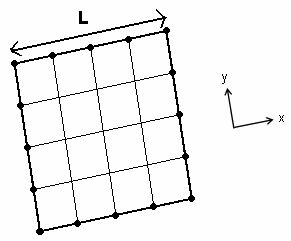Thickness: th = 50 mm

### Analysis Specifications

 Young Modulus (material): E = 200 GPa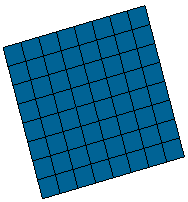Poisson's Ratio (material): ν = 0.3 Density: ρ = 8000 kg/m3 Restraints: Tx = Ty = Rz = 0 at all nodes Tz = 0 along all four edges Rx = 0 along edges x = 0 and x = 10000 mm Ry = 0 along edges y = 0 and y = 10000 mm Loads: F0 = 100 N/m2 over whole plate Damping: d = 2 % in all 16 modes used Time step: t = 0.002 s

##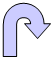Results

You will find here the results for different finite elements:

 Type of values Reference solution Values Linear triangle shell (TR3) 64 x 64 elements Parabolic triangle shell (TR6) 32 x 32 elements Linear quadrangle shell (QD4) 32 x 32 elements Parabolic quadrangle shell (QD8) 16 x 16 elements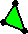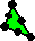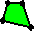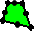Values Error [%] Values Error [%] Values Error [%] Values Error [%] Peak displacement [mm] at t=0.210s 3.523 3.444 2.24 3.445 2.21 3.451 2.04 3.446 2.19 Peak stress [MPa] 2.484 2.221 10.59 2.217 10.75 2.251 9.38 2.234 10.06 Static displacement [mm] 1.817 1.774 2.37 1.775 2.31 1.776 2.26 1.775 2.31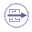To Perform the Test:

The Transient_forced_vibration_of_a_simply_supported_thin_square_plate.CATAnalysis document presents a complete analysis of this case, computed with a mesh formed of linear quadrangle elements (QD4).

To compute the case with parabolic quadrangle (QD8), linear triangle (TR3) and parabolic triangle (TR6) elements, proceed as follow:

1. Open the CATAnalysis document.

2. In the Advanced Meshing Tools workbench, replace the mesh specifications as indicated above.

3. In the Generative Structural Analysis workbench, compute the case.